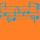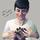## General Question# Can someone help me with this Python problem?

Asked by dxs (15160) February 10th, 2015

I’m learning Python in a computer programming class. We looked at how to make exponents (x**y). I want to find the highest number that Python can produce, so I made a function to imput a number into a 10-based exponent. Basically, f(x)=10^x
Here’s what I wrote:

`1 import math`
`2 import time`
`3 number = int(input(‘number, please:’))`
`4 start = time.time()`
`5 alewife = 10**number`
`6 elapsed = time.time()-start`
`7 print(“Ten to the power of ” + str(number) + ” is ” + str(alewife))`
`8 print(“This computation took ” + str(elapsed) + ” seconds”)`

I’m on Pycharm. On the bottom of the screen, I get the “Number, please:” prompt. When I enter in a number, it tells me 10 to the power of that number, and how long the computation took.
Now here’s the first problem: The time it takes to compute it is not the same as the time it takes to display it. It says it takes only a few seconds to compute it when it takes a few minutes to display it.
I’m between 106 and 107, since 107 seems too big (it took too long to respond). I did 106.5, 106.25, etc., up to the most recent 106.484375.
Here’s the second problem: With this number, it gave me an answer, but it didn’t print the second line (which tells me how long it took to compute). My guess was that it’s because I ran out of characters.
Any help is appreciated, thanks.

Observing members: 0Composing members: 0## 10 AnswersPython is unique among mainstream modern programming languages because it doesn’t have integer overflow.

Here’s what I mean by that. In most other programming languages, there is a distinct “maximum integer” that can be displayed.

That is, if you try to display too high of an integer (beyond that which can fit in 32 bits, or 2^31–1), the integer will actually wrap around back to the minimum integer, or -2^31. That is, 2^-31–1 + 1 = -2^-31, even though this is mathematically fallacious. This happens because there simply isn’t enough memory to hold a larger number.

But Python is different. Python keeps allocating more and more memory to your integer to accommodate its larger size. But there is still a limit – albeit a ridiculous one – the amount of memory available to your program. And that’s the limit I expect you’re hitting. The integer is eating up all the available memory and the program is unable to continue.

Mariah (25876)“Great Answer” (3) Flag as…I see now that the description has some format errors. There are supposed to be carets in that lower paragraph, where the small text beings and ends. That paragraph had explained that 10 to the 7th power took too long (meaning I ran out of patience), so I stopped there. Oh, and you may have figured this out but I’m talking about 10 to the (10 to the 7th) when I was saying 10 to the 7th power. My details were misleading—I really don’t want to find a specific “maximum” number, just a function that depicts the time it takes to compute certain exponents. Then I can estimate a graph and perhaps estimate further times by using a differential equation or something, I don’t know, I’m dabbling in math.
So anyways, that’s what I was saying when I stopped at 10 to the 7th and started using powers between 10 to the 6th and 10 to the 7th. If I can get computing times that take more than, say, 1 second, but less than 1 or even 10 minutes, I can get a significant graph.

dxs (15160)“Great Answer” (0) Flag as…Ahhhh I thought you meant 10 to the 107th power. 10^7 should not hit Python’s memory limit. I’d have to see your exact code to troubleshoot what the problem might be.

Mariah (25876)“Great Answer” (1) Flag as…I ran your program exactly as you wrote it using the regular Python interpreter. In the versioin of Python that I am using, the expression x**y expects an integer value for y and will give an error if you try to use a floating point number.

The program did not even break into a sweat. I was able to enter numbers greater than 100 and the program immediately returned the answer and gave 0 seconds. A number like 10100 is extraordinarily large, but only requires a few hundred bytes to represent. The number of multiplications required is also not that large. Computing xn requires around log n base 2 multiplications. I can explain why this is so if you are interested.

I am not familiar with Pycharm. I will do a Google search to see if it is something I can work with online.

LostInParadise (28710)“Great Answer” (1) Flag as…What I said was not exactly right. The error I got for not entering an integer was for the int() part of the read statement. I can use non-integer y values for x**y. I wonder if Python treats integer values of y differently in the way it computes the expression. I can write 10**106.4 and the program returns immediately.

LostInParadise (28710)“Great Answer” (1) Flag as…@Mariah That’s because it’s [10 to the (10 to the 7th)]. Or, 10 to the 10000000th power. The formatting in the details took out the the carets and my correction just made it more complicated.
@LostInParadise I’m using Python 3.4. When I typed in the exponent at the “number, please:” prompt, I was entering in the whole value (100000, 10000000, etc.). I don’t know how to do this without PyCharm because when I open up Python, it just gives me the three arrow prompt. What I want it a prompt saying “number, please:”. Oh, and if you didn’t. you may want to take out the line numbering.

dxs (15160)“Great Answer” (0) Flag as…For the very large numbers you are working with, I can see Python getting into trouble. If you are trying to print out millions of digits, it is going to take a while. I set a variable x to 10 to the 10 to the 7th. It took a few seconds. To test it I took x mod 3 (x % 3 in Python). This correctly came back as 1. When I tried x mod 11, it gave back 1, which is also correct. I just tried this on the Wolfram Alpha site and the program is still running. I will let it continue to see what happens. My guess is that it will eventually time out.

If you want to run your code on Python, put it in a function and then call the function. I don’t know if you learned about functions yet. I did remove the line numbers from your code. It would never have been able to run otherwise.

LostInParadise (28710)“Great Answer” (1) Flag as…WOW I only just noticed that the exact code is literally in the question details…..d’oh. I’ll look again later when I have more time unless @LostInParadise has already answered you to your satisfaction.

Mariah (25876)“Great Answer” (1) Flag as…I found out how to get the results I want! To recap, I am looking for how long it takes Python to compute the results, not the actual, numerical answer (i.e. the 10^(10^x)). The answer was simple: take out line 7. It solves both of the assumed problems.
Here’s a summary of my process that I handed in to my professor:
http://issuu.com/dxs3/docs/exponent
(Click to zoom in; Skip down to the second page to see the graph of the time it took for Python to compute each value).

Right now, I’m working on a program where if I input a prime number, it gives me how many digits in the repetend of 1 over that prime number. I’m doing this because I was analyzing the relationship between the value of the prime number and the digits in its repetend, and I noticed that many relations are such that the digit’s value is one less than the prime number’s value.
If that makes no sense to you, here’s the pattern:
1/7 has 6 digits in its repetend.
1/17 has 16 digits in its repetend.
1/19 has 18 digits in its repetend.
½3 has 22 digits in its repetend.

See how 6 is one less than 7, 16 is one less than 17, etc…
I want to see if this plays out in bigger primes. I’ve already run into a problem: Python isn’t accurate in its calculations! For instance, f I tell it to divide 1 by 3, it spits out something like “0.333333333333333029834”. That random number vomit at the end has something to do with Python not being able to express certain values in binary.
To avoid this, I’m trying to write a program that sums numbers so I can make the summation of a geometric series such as 3*(1/10)^n (which is ⅓). I’m having trouble doing this, though.

Geez, I really digressed. Anyways I hope you at least found it interesting.

dxs (15160)“Great Answer” (0) Flag as…It is interesting that Python can perform such large calculations in a relatively short time. The length of the repetend of 1 over a prime p always divides p-1. In the case of ⅓, this works since the length 1 divides 2. I have a book at home that gives a nice explanation at an elementary level. Unfortunately, the title slips my mind. When I get back home, I will give it to you.

You can work with fractions in Python by importing the fractions library. If you want to work with ⅓, just write x=Fraction(1,3). You can do arithmetic with the fractions just like you would with regular numbers. You can do x+y or x**2. The answer will always be a fraction in the form (n,d) with numerator n and denominator d. To get the parts of the fraction, write x.numerator and x.denominator For more information, check out the Python fraction library information

LostInParadise (28710)“Great Answer” (0) Flag as…## Answer this questionor

#### Join

to answer.

This question is in the General Section. Responses must be helpful and on-topic.

Your answer will be saved while you login or join.
Have a question? Ask Fluther!
What do you know more about?
or
Knowledge Networking @ Fluther